# Orders of magnitude (one cubic millimetre to one cubic metre)

The following is a table of objects with volumes or capacities of between one cubic millimetre and one cubic metre.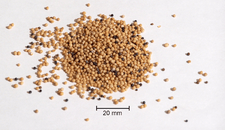mustard seeds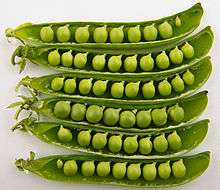peas in pods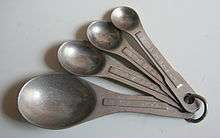measuring spoons of 1 tablespoon and 1, 12 and 14 teaspoon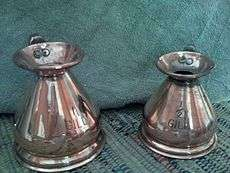copper measuring jugs of 1 and 12 gill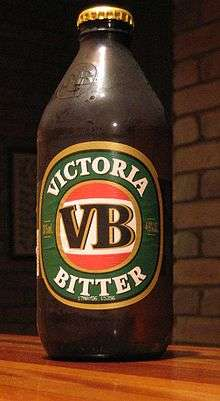375 ml stubbie of beer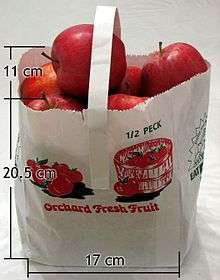a 12-peck apple bag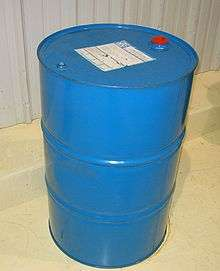a standard 200-litre
(55 US or 44 imp gal) drum
volume (m3) example
1×10−9 one cubic millimetre or one microlitre
4×10−9 a large grain (granule) of sand (2.0 mm diameter, 95 milligrams)
4×10−9 volume of a mustard seed of 2-millimetre diameter
2×10−8 volume of a small grain of rice 2 mm wide by 5 mm long
5.92×10−8 one imperial minim
6.16×10−8 one US minim
7×10−8 volume of a large grain of rice 3 mm wide by 12 mm long
2×10−7 average volume of a pea
1×10−6 one cubic centimetre or one millilitre
1.18×10−6 one imperial fluid scruple
1.23×10−6 one US fluid scruple
1.80×10−6 one sai
3.55×10−6 one imperial fluid drachm
3.70×10−6 one US fluid dram
3.55–5×10−6 one teaspoon
1.14×10−5 one ligula
1.42–2.0×10−5 one tablespoon
1.639×10−5 one cubic inch
1.80×10−5 one shaku
2.84×10−5 one imperial fluid ounce
2.96×10−5 one US fluid ounce
3.5×10−5 average amount of blood lost by a woman during menstruation
4.5×10−5 one cyathus
6.8×10−5 one acetabulum
1.18×10−5 one US gill
1.36×10−4 one quartarius
1.42×10−5 one imperial gill
1.80×10−4 one (a common size for serving sake)
2.73×10−4 one Roman hemina or cotyla
3.3–3.75×10−4 volume of stubby or steinie of beer (Europe–330 ml, Canada–341 ml, Japan–350 ml, US–355 ml, Australia–375 ml)
4×10−4 rough volume of the human urinary bladder
4.73×10−4 one US liquid pint
5.46×10−4 one sextarius
5.51×10−4 one US dry pint
5.68×10−4 one imperial pint
7.5×10−4 the most common volume for wine and liquor bottles, also the size of an Australian long neck of beer; sometimes called a 'fifth' in the United States for its approximation to the once-common one-fifth-gallon bottle
9.46×10−4 one US liquid quart
1×10−3 one cubic decimetre or one litre
1.000028×10−3 volume of 1 kilogram of distilled water (at the temperature of maximum density (3.98 °C or 39.16 °F) and standard atmospheric pressure (101.325 kPa))
1.10×10−4 one US dry quart
1.14×10−3 one imperial quart
1.0–8.2×10−3 typical range of automobile engine displacements
1.4×10−3 human brain cavity
1.80×10−3 one shō (formerly a common sake-bottle size)
3.27×10−3 one congius
3.8×10−3 one US liquid gallon
4.36×10−3 one semimodius
4.40×10−3 one US dry gallon
4.5×10−3 one imperial gallon
5×10−3 approximate volume of human blood
6×10−3 average total volume of the male lungs
8.73×10−3 one modius
8.81×10−3 one US peck
9.09×10−3 one imperial peck
1.31×10−2 one urna
1.80×10−2 one to
1.85–3.6×10−2 one Ancient Greek amphora
2.62×10−2 one Roman amphora
3.4×10−2 one French amphora
2.83×10−2 one cubic foot
3.52×10−2 one US bushel
3.64×10−2 one imperial bushel
3.7–4.2×10−2 one firkin
6.8–6.9×10−2 one rundlet
7.1×10−2 average volume of an adult human
7.4–8.3×10−2 one kilderkin
9.55×10−2 one US barrel for cranberries
1.16×10−1 one US dry barrel
1.17×10−1 one US beer barrel, 31 US gallons
1.19×10−1 one US fluid barrel (apart from oil or beer), 31.5 US gallons
1.59×10−1 one oil barrel, 42 US gallons, about one tierce (158–160 l)
1.64×10−1 one imperial barrel, 36 imperial gallons
1.80×10−1 one koku
2×10−1 standard drum size used for shipping bulk cargo